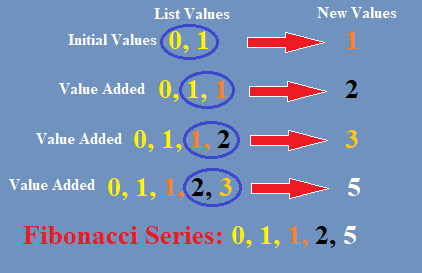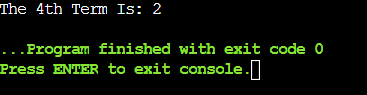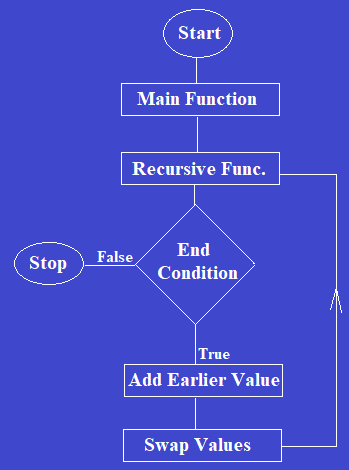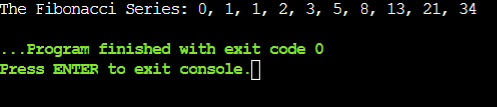# Fibonacci Series Program In C

Do you know what Fibonacci Sequence is? Have you ever thought about how one mathematical concept is aligned with the C programming concept?

In this article, we will discuss all the necessary elements of the Fibonacci Sequence. We will start our discussion with the Mathematical background of the Fibonacci sequence.

Later we will move to the development of the Fibonacci series in C programming language.

### What Is The Mathematical Background Of The Fibonacci Sequence?

In Mathematics, we are all aware of the presence of different series & sequences. One of the important series is Fibonacci Series. The Fibonacci Row is developed with the addition of the previous value along with the present value.Here, the first two numbers are Zero & One. The Concept says that the next number will be the addition of these two numbers. In this way, the complete series goes on. We can make a Fibonacci Series with up to 5th term:

#### The Fibonacci Series Up To The 5th Term: 0, 1, 1, 2, 3.

``````Process To Get The nth Term In Series:
Fn = Fn-1 + Fn-2 [F0 will be 0 & F1 will be 1]``````

In Mathematics, the Fibonacci Series follows one property. The property is mentioned above. Along with that, there is a formula present to get the direct term without going through the series. Just put the term number in the formula.

``Formula To Get The nth Term Directly Without Series: {[(√5 + 1)/2] ^ (n-1)} / √5``

### How To Get Direct Term Of Fibonacci Series In C Program?

To get the direct term without involving inside any loop or recursive call function case, we can just implement the formula for Fibonacci Series. Implementation of the formula to get the nth term will help to reduce the time & space complexity of the program. We can understand that programming with mathematics can be a little difficult to understand, so you can always hire CodingZap experts if you need any assistance with your C homework.

#### Code To Implement Formula Of Fibonacci Series In C:

``````#include<stdio.h>
#include<math.h> // Importing Package To Use Mathematical Functions
# define one (sqrt(5) + 1) / 2 // Defining The 1st Part Formula

int main ()
{
int n = 4; // Term Value
double sum = round(pow(one, n-1) / sqrt(5)); // Implementing Formula Using Variable Sum
printf("The %dth Term Is: %.0lf", n, sum); // Printing Value Using Term & Sum Variable
return 0;
}``````

Steps Of The Program:

1. First, we have imported one necessary library that will be used to handle the mathematical terms.
2. Now, we have defined one variable that will be used to implement the first part of the formula.
3. Now, the term value will be provided inside the program. And the remaining formula will be implemented.
4. We will print the value along with the term number that is taken inside the program.

Let us try to find out the output of the above code. It will help to know the implementation process of the formula to get Fibonacci Numbers.

#### Output:From the above output, we can see that the direct term number is coming. And in this case, we have not implemented the while loop & recursive calling function. Such a program helps to get a specific term without increasing time & space complexity.

### What Are The Methods To Implement Fibonacci Series Program?

There are two possible methods present to implement the Fibonacci Numbers in the C programs. In one case, the traditional loop will be used. And in another case, the precision method is used.

1. Fibonacci Series Using Loops
2. Fibonacci Series Using Recursion

Let us look at them one by one briefly.

### How To Implement Fibonacci Series Program Using Loop Concept?

The C Fibonacci Series can be implemented with two types of loops. One is the While Loop & another is the For Loop. Using the For Loop is much more convenient as we don’t need to modify the value separately inside the program. Loops in Python are used in an interesting way to make the condition satisfied. Loops

First, we will implement the Fibonacci program using the for loop. Later, using the While looping concept.#### Code To Calculate Fibonacci Series In C Using For Loop Concept:

``````#include<stdio.h>
int main() // // Main Function To Print Fibonacci Series Program In C
{
int a1=0, a2=1; // Initial First Two Terms
int n=5; // Term Value
int a3, i;
// int fibonacci = 0;

printf("The Fibonacci Series: %d, %d", a1, a2); // Printing Initial Values
for(i=2; i<n; ++i) // Loop Starts From Third Number
{
a3 = a1 + a2;
printf(", %d", a3); // Printing Values
a1 = a2;
a2 = a3;
}
return 0;
}    ``````

Steps Of The Program:

1. In the above example, we have initialized the previous numbers of the series. Those are the starting values i.e. Zero & One.
2. Now, the integer third term will be declared to get the values from the loop. Now, the for loop will be implemented.
3. The implementation of the for loop will be done from the third term because the first two terms are already declared. The for loops will print the numbers preceding those two initial values.
4. Inside that for loop, we will use the print statement to display Fibonacci Sequence.

Let us try to find out the output of the above code. It will help to know the implementation process of the for loop to get Fibonacci Numbers.

#### Output:From the above output, we can see that the Fibonacci Sequence is printed using the C programming language. And the series is printed up to the particular number of terms mentioned inside the program.

Code To Calculate Fibonacci Sequence In C Using While Loop Concept:

``````#include<stdio.h>
int main()
{
int a1=0, a2=1, n=15; // Initial Values & Term Value
int a3, i=2;

printf("The Fibonacci Series: %d, %d", a1, a2); // Printing Initial Values
while (i<n) // Loop Starts From Third Number
{
a3 = a1 + a2;
printf(", %d", a3); // Printing Values
a1 = a2;
a2 = a3;
++i;
}
return 0;
}``````

Steps Of The Program:

1. In this program also, there is a need to declare the initial values & the term value to get the Fibonacci Sequence.
2. Now, the while loops will be used. Inside the loop, all the operations will be done. We should get the summation values by iterating each time.
3. Now, the modification of the variable will be done separately as it is a While loop. And the values will be printed.

Let us try to find out the output of the above code. It will help to know the implementation process of the While loops to get Fibonacci Number.

#### Output:From the above output, we can see that the While loop implementation also works the same as the For loop implementation process. In the above case, the Fibonacci Sequence of up to fifteen terms is printed successfully.

### How To Implement Fibonacci Series Using Recursion?

To get Fibonacci digits using the recursion Fibonacci Series, you should first understand the recursion method. In the recursion method, one function calls itself more times & one ending condition is provided there to terminate that. If you wish to know about recursion, you can read out our article on recursion in C++ to make things more clear.#### Code To Implement Recursion Fibonacci Series Up To Ten Terms:

``````#include<stdio.h>
void print(int n) // Function To Print Fibonacci Series Program In C
{
static int a1=0, a2=1, a3;  // Initial Two Digits
if(n>0) // Conditional Ending Statement
{
a3 = a1 + a2; // Statement For Registered Marks
a1 = a2;
a2 = a3;
printf(", %d", a3); // Printing Number Of Terms
print(n-1); // Recursive Calling Function
}
}
int main()
{
int n = 10; // Term Value
printf("The Fibonacci Series: 0, 1"); // Printing Initial Values
print(n-2); // Calling Function To Create Recursion Tree
return 0;
} ``````

Steps Of The Program:

1. In the above program, we have seen the term value is taken inside of the int main() function.
2. Now, the previous two values will be printed in the program. These are the initial values that are needed to start the program.
3. Now, the function will be called from the int main() function. And inside the function, one conditional statement is used.
4. Inside that conditional statement, we will display Fibonacci Sequence using the print statement. This function will be called recursively to print the remaining terms.
5. When the condition becomes false, the function will be finished along with the program.

Let us try to find out the output of the above code. It will help to know the implementation process of the Recursive Method to get Fibonacci Digit.

#### Output:From the output, we can see that the along with the first two terms, further terms are also printed in the Fibonacci Series. So, the Fibonacci Series in C program is implemented without any error.

### Conclusion:

As we saw it is very important to know the Fibonacci Sequence in the C programming language.

The Fibonacci Series might be a concept from Mathematics, but it has one important role in the project management institute. Such problems help to grow the logical thinking & programming skills of the students.

It is advisable to clear the basic knowledge of the C programming language before moving to this topic. Though the problem with Fibonacci Series is much easier than any other problem, having good basic knowledge helps to make the concept clearer.

f you ever find yourself in need of help with programming homework, don’t hesitate to reach out for assistance. At CodingZap, we have a team of ace coders ready to lend a hand and ensure you excel in your programming assignments.